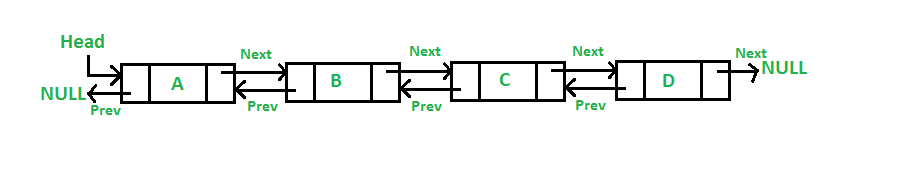• Last Updated : 23 Aug, 2019

A Doubly Linked List is a list that contains links to both next and previous nodes. A Doubly Linked List (DLL) contains an extra pointer, typically called previous pointer, together with next pointer and data which are there in singly linked list. Unlike singly linked lists where traversal is only one way, doubly linked lists allow traversals in both ways.Following are the important terms to understand the concept of doubly linked list:

• Link: Each node of a linked list can store a pointer to another element called a Link.
• Next: Each node of a linked list contains a link to the next node called Next.
• Prev: Each node of a linked list contains a link to the previous node called Prev.

You may refer to article on Doubly Linked List ( Introduction ) for understanding the doubly linked list in details.

The SplDoublyLinkedList class is a PHP Library class which provides the main functionalities of a doubly linked list in PHP.

Below are some of the basic functions of the SplDoublyLinkedList class:

• push(): This function is used to insert a new node at the end of a doubly linked list.
Syntax:

```list_name.push(value);
```

This will push the value at the end of list list_name.

• pop(): This function is used to delete a node from the end of doubly linked list.
Syntax:

```list_name.pop()
```

This will delete the last node from the list list_name.

• top(): This function is used to pick the node at the end of the doubly linked list.
Syntax:

```list_name.top()
```

This will return the last node from the list list_name.

• bottom(): This function is used to pick the node at the beginning of the doubly linked list.
Syntax:

```list_name.bottom()
```

This will return the node present at the beginning of the list list_name.

• add(): This function is used to insert a new value at a specified index in a Doubly linked list, this also causes shuffling of the previous value at that index (and all subsequent values) up through the list.
Syntax:

```list_name.add('index', "value");
```

This will add the value at position index in the list list_name.

• count(): This function is used to count the number of elements present in given Doubly linked list.
Syntax:

```list.count()
```

This will return the count of total number of elements present in the list list_name.

Below program illustrate the above functions to create and use a doubly linked list:
Program:

 `push(``'geeks'``);``\$dlist``->push(``'for'``);``\$dlist``->push(``'practice'``);`` ` `// Displaying the list``echo` `"Original List : "``;``for` `(``\$dlist``->``rewind``(); ``\$dlist``->valid(); ``\$dlist``->next()) {``    ``echo` `\$dlist``->current().``" "``;``}`` ` `// Deleting element from the end of the list``\$dlist``->pop();`` ` `// Adding a new element at specific index``// Add 'code' at index 2``\$dlist``->add(2, ``"code"``);`` ` `// Displaying the updated list``echo` `"\n\nUpdated List : "``;``for` `(``\$dlist``->``rewind``(); ``\$dlist``->valid(); ``\$dlist``->next()) {``    ``echo` `\$dlist``->current().``" "``;``}`` ` `// Printing the count of nodes``echo` `"\n\nCount = "` `. ``\$dlist``->``count``() . ``"\n"``;`` ` `// Printing the node at top of the list``echo` `"Top = "``. ``\$dlist``->top() . ``"\n"``;`` ` `// Printing the node at bottom of the list``echo` `"Bottom = "` `. ``\$dlist``->bottom() . ``"\n"``;`` ` `?>`

Output:

```Original List : geeks for practice

Updated List: geeks for code

Count = 3
Top = code
Bottom = geeks
```

My Personal Notes arrow_drop_up